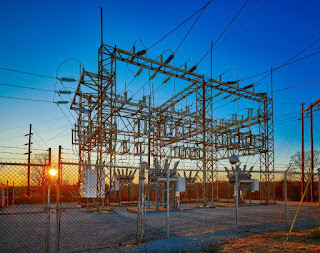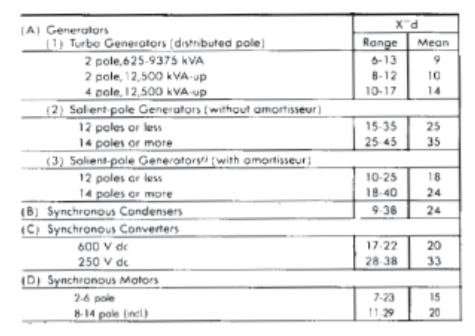Substation

When determining the magnitude of short-circuit currents, it is extremely important that all sources of short-circuit current he considered and that the reactance characteristics of these sources be known.

There are three basic sources of short-circuit current:

1. Generators
2. Synchronous motors and synchronous condensers
3. Induction motors

Generators
Generators are driven by turbines, diesel engines, water wheels, or other types of prime movers. When a short circuit occurs on the circuit fed by a generator, the generator continues to produce voltage because the field excitation is maintained and the prime mover drives the generator at substantially normal speed.

The generator sets, however, have various base ratings, and so there is a need to convert from the alternator base to the generator set base.

The conversion is accomplished using the following formula:

For example, suppose you want to find the sub transient reactance (X"d ) in Per-Unit for the 105°C rises, The 480-volt alternator on a 750 kW DFHA generator set. From the generator set specification sheet, S-1132, the alternator data-sheet is ADS-311. ADS-311 shows a sub-transient reactance of 0.16 P.U. based on the 125°C alternator base rating of 1300kVA. The base kVA rating of the 750DFHA is 938 kVA.

Using the formula, calculate X"d on the generator set base as follows:

The generated voltage produces a shortcircuit current of a large magnitude which flows from the generator (or generators) to the short circuit. This flow of short-circuit current is limited only by the impedance of the generator and of the circuit between the generator and the short circuit. For a short circuit at the terminals of the generator, the current from the generator is limited only by its own impedance.Typical per unit reactance values for generators ranging from 40 to 2000 kW.

Synchronous Motors
Synchronous motors are constructed substantially like generators, as such, it has a field excited by direct current and a stator winding in which alternating current flows. Normally, synchronous motors draw a-c power from the line and convert electric energy to mechanical energy. However, the design of an asynchronous motor is so much like that of a generator that electric energy can be produced just as in a generator, by driving the synchronous motor with a prime mover.

The synchronous motor then becomes a generator and delivers shortcircuit current for many cycles after the short circuit occurs on the system. The amount of current depends upon the horsepower, voltage rating, and reactance of the synchronous motor and the reactance of the system to the point of a short circuit.

Induction Motors
The inertia of the load and rotor of an induction motor has exactly the same effect on an induction motor as on an asynchronous motor; i.e., it drives the motor after the system short circuit occurs. There is one major difference. The induction motor has no d-c field winding, but there is a flux in the induction motor during normal operation. This flux acts like flux produced by the d-c field winding in the synchronous motor.Normally motors draw load current from the source or utility system but produce shortcircuit current when a short circuit occurs in the load.

The field of the induction motor is produced by induction from the stator rather than from the d-c winding. The rotor flux remains normal as long as the voltage is applied to the stator from an external source. However, if the external source of voltage is removed suddenly, as it is when a short circuit occurs on the system, the flux in the rotor cannot change instantly. Since the rotor flux cannot decay instantly and the inertia drives the induction motor, a voltage is generated in the stator winding causing a short-circuit current to flow to the short circuit until the rotor flux decays to zero.A. Percent values on machine KVA ratingANSI Standard impedances and interrupting duty multipliers

Note: X" for induction motor groups >50 HP and <50 HP are typically assumed equal to 16.7%. Using the impedance multipliers, this corresponds to an equivalent motor contribution of 3.6 to 4.8 times the full load current.

Transformers
Transformers are often spoken of as a source of short-circuit current. Strictly speaking, this is not correct, for the transformer merely delivers the short-circuit current generated by generators or motors ahead of the transformer. Transformers merely change the system voltage and magnitude of current but generate neither. The short-circuit current delivered by a transformer is determined by its secondary voltage rating and reactance, the reactance of the generators and system to the terminals of the transformer, and the reactance of the circuit from the transformer to the short circuit.

The reactance of Rotating Machines

The three reactances can be briefly described as follows:
1. Subtransient reactance, is the apparent reactance of the stator winding at the instant short circuit occurs, and it determines the current Row during the first few cycles of a short circuit.
2. Transient reactance, is the apparent initial reactance of the stator winding if the effect of all amortisseur windings is ignored and only the field winding considered. This reactance determines the current following the period when sub transient reactance is the controlling value. Transient reactance is effective up to 0.5 seconds or longer, depending upon the design of the machine.
3. Synchronous reactance, is the apparent reactance that determines the current flow when a steady-state condition is reached. It is not effective until several seconds after the short circuit occurs; consequently, it has no value in short-circuit calculations for the application of circuit breakers, fuses, and contactors but are useful for relay-setting studies.

Reference:
• Industrial Power System Handbook, Donald Beeman | McGRAW-HILL BOOK COMPANY, INC.
• Calculating generator reactances, Timothy A. Loehlein